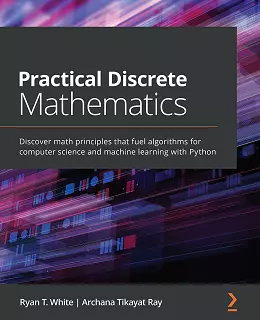# Practical Discrete Mathematics### eBook Details:

• Paperback: 330 pages
• Publisher: WOW! eBook (February 22, 2021)
• Language: English
• ISBN-10: 1838983147
• ISBN-13: 978-1838983147

### eBook Description:

Practical Discrete Mathematics: A practical guide simplifying discrete math for curious minds and demonstrating its application in solving problems related to software development, computer algorithms, and data science

Discrete mathematics deals with studying countable, distinct elements, and its principles are widely used in building algorithms for computer science and data science. The knowledge of discrete math concepts will help you understand the algorithms, binary, and general mathematics that sit at the core of data-driven tasks.

Practical Discrete Mathematics is a comprehensive introduction for those who are new to the mathematics of countable objects. This book will help you get up to speed with using discrete math principles to take your computer science skills to a more advanced level.

As you learn the language of discrete mathematics, you’ll also cover methods crucial to studying and describing computer science and machine learning objects and algorithms. The chapters that follow will guide you through how memory and CPUs work. In addition to this, you’ll understand how to analyze data for useful patterns, before finally exploring how to apply math concepts in network routing, web searching, and data science.

• Understand the terminology and methods in discrete math and their usage in algorithms and data problems
• Use Boolean algebra in formal logic and elementary control structures
• Implement combinatorics to measure computational complexity and manage memory allocation
• Use random variables, calculate descriptive statistics, and find average-case computational complexity
• Solve graph problems involved in routing, pathfinding, and graph searches, such as depth-first search
• Perform ML tasks such as data visualization, regression, and dimensionality reduction

By the end of this Practical Discrete Mathematics book, you’ll have a deeper understanding of discrete math and its applications in computer science, and be ready to work on real-world algorithm development and machine learning.